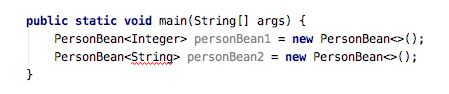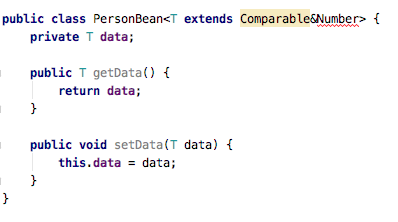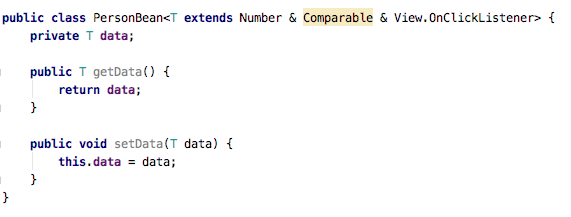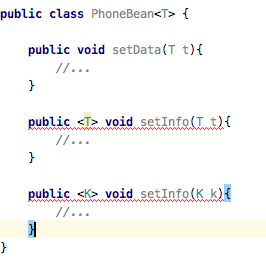# Java泛型那些事儿(一)

## 一、为什么我们需要泛型？

``````public class CountBean {

public boolean compareInt(int x, int y) {
return x > y;
}

public boolean compareFloat(float x, float y) {
return x > y;
}

public static void main(String[] args) {
//示例代码一
CountBean countBean = new CountBean();
boolean result1 = countBean.compareInt(2, 3);
boolean result2 = countBean.compareFloat(2.4f, 3.6f);

System.out.println("result1=" + result1);
System.out.println("result2=" + result2);

//示例代码二
List list = new ArrayList();
}

}
``````

``````public class CountBean {

public <T extends Number & Comparable> boolean compare(T x, T y) {
/**
* x>y 返回1
* x=y 返回0
* x<y 返回-1
*/
return x.compareTo(y) == 1;
}

public static void main(String[] args) {
//示例代码一
CountBean countBean = new CountBean();
boolean result1 = countBean.compare(2, 3);
boolean result2 = countBean.compare(2.4f, 3.6f);

System.out.println("result1=" + result1);
System.out.println("result2=" + result2);

//示例代码二
List<String> list = new ArrayList();
//指定了List的泛型类型为String，所以传入数据类型会报错
}

}
``````

## 二、泛型类、泛型接口、泛型方法解析

1. 泛型类的定义
泛型类的定义和普通的类定义没有很大的不同，只是在类名后面添加了<T>，其中T可以是其他的任意字母。大概有以下几种情况：

``````public class PersonBean<T> {
private T data;

public T getData() {
return data;
}

public void setData(T data) {
this.data = data;
}
}
``````

``````public class PersonBean<T extends Number> {
private T data;

public T getData() {
return data;
}

public void setData(T data) {
this.data = data;
}
}
``````

``````public class PersonBean<T extends Number & Comparable> {
private T data;

public T getData() {
return data;
}

public void setData(T data) {
this.data = data;
}
}
``````

``````public class PersonBean<K,T extends Number & Comparable> {
private T data;

public T getData() {
return data;
}

public void setData(T data) {
this.data = data;
}
}
``````1. 泛型接口的定义
泛型接口的定义和普通接口的定义差不多，规则和上面的泛型类一样，只是需要将关键字class改成interface。泛型接口也可以被别的泛型接口继承，扩展更多的功能。
2. 泛型方法的辨析
泛型方法不是特指定义在泛型类的方法。像下面的这种都不是泛型方法：
``````public T getData() {
return data;
}

public void setData(T data) {
this.data = data;
}
``````

``````public <T> T test(T t) {
return t;
}

public <K, T> T test(K k, T t) {
return t;
}

public <T extends Number> T test(T t) {
return t;
}

public <T extends View & Comparable> T test(T t) {
return t;
}

public <K, T extends View & Comparable> T test(K k, T t) {
return t;
}
``````

``````public class PhoneBean<T> {

public void setData(T t){
//...
}

public <T> void setInfo(T t){
//...
}

public <K> void setUser(K k){
//...
}
}
``````